# Subnetting in Networking | Subnetting Examples

## Subnetting in Networking-

In networking,

• The process of dividing a single network into multiple sub networks is called as subnetting.
• The sub networks so created are called as subnets.

## Example-

Following diagram shows the subnetting of a big single network into 4 smaller subnets-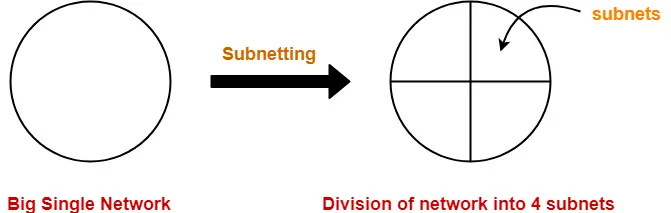The two main advantages of subnetting a network are-

• It improves the security.
• The maintenance and administration of subnets is easy.

## Subnet ID-

• Each subnet has its unique network address known as its Subnet ID.
• The subnet ID is created by borrowing some bits from the Host ID part of the IP Address.
• The number of bits borrowed depends on the number of subnets created.

## Types of Subnetting-

Subnetting of a network may be carried out in the following two ways-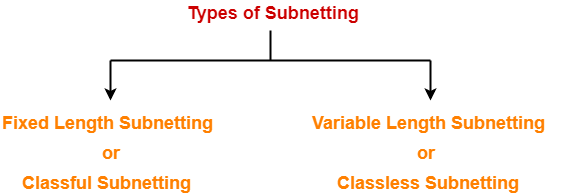1. Fixed Length Subnetting
2. Variable Length Subnetting

## 1. Fixed Length Subnetting-

Fixed length subnetting also called as classful subnetting divides the network into subnets where-

• All the subnets are of same size.
• All the subnets have equal number of hosts.
• All the subnets have same subnet mask.

## 2. Variable Length Subnetting-

Variable length subnetting also called as classless subnetting divides the network into subnets where-

• All the subnets are not of same size.
• All the subnets do not have equal number of hosts.
• All the subnets do not have same subnet mask.

## Subnetting Examples-

Now, we shall discuss some examples of subnetting a network-

## Example-01:

Consider-

• We have a big single network having IP Address 200.1.2.0.
• We want to do subnetting and divide this network into 2 subnets.

Clearly, the given network belongs to class C.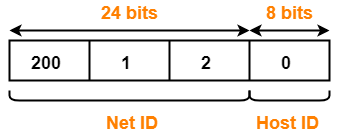For creating two subnets and to represent their subnet IDs, we require 1 bit.

So,

• We borrow one bit from the Host ID part.
• After borrowing one bit, Host ID part remains with only 7 bits.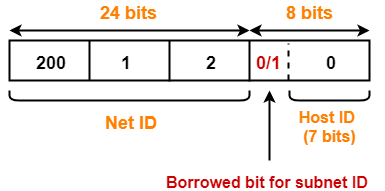• If borrowed bit = 0, then it represents the first subnet.
• If borrowed bit = 1, then it represents the second subnet.

IP Address of the two subnets are-

• 200.1.2.00000000 = 200.1.2.0
• 200.1.2.10000000 = 200.1.2.128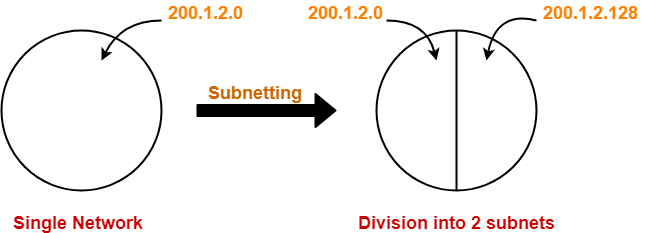## For 1st Subnet-

• IP Address of the subnet = 200.1.2.0
• Total number of IP Addresses = 27 = 128
• Total number of hosts that can be configured = 128 – 2 = 126
• Range of IP Addresses = [200.1.2.00000000, 200.1.2.01111111] = [200.1.2.0, 200.1.2.127]
• Direct Broadcast Address = 200.1.2.01111111 = 200.1.2.127

## For 2nd Subnet-

• IP Address of the subnet = 200.1.2.128
• Total number of IP Addresses = 27 = 128
• Total number of hosts that can be configured = 128 – 2 = 126
• Range of IP Addresses = [200.1.2.10000000, 200.1.2.11111111] = [200.1.2.128, 200.1.2.255]
• Direct Broadcast Address = 200.1.2.11111111 = 200.1.2.255

## Example-02:

Consider-

• We have a big single network having IP Address 200.1.2.0.
• We want to do subnetting and divide this network into 4 subnets.

Clearly, the given network belongs to class C.For creating four subnets and to represent their subnet IDs, we require 2 bits.

So,

• We borrow two bits from the Host ID part.
• After borrowing two bits, Host ID part remains with only 6 bits.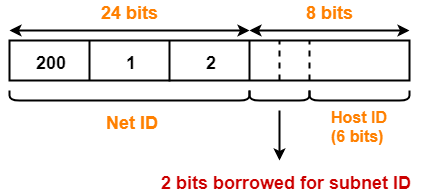• If borrowed bits = 00, then it represents the 1st subnet.
• If borrowed bits = 01, then it represents the 2nd subnet.
• If borrowed bits = 10, then it represents the 3rd subnet.
• If borrowed bits = 11, then it represents the 4th subnet.

IP Address of the four subnets are-

• 200.1.2.00000000 = 200.1.2.0
• 200.1.2.01000000 = 200.1.2.64
• 200.1.2.10000000 = 200.1.2.128
• 200.1.2.11000000 = 200.1.2.192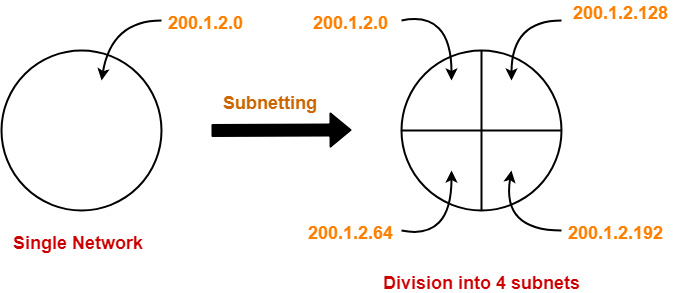## For 1st Subnet-

• IP Address of the subnet = 200.1.2.0
• Total number of IP Addresses = 26 = 64
• Total number of hosts that can be configured = 64 – 2 = 62
• Range of IP Addresses = [200.1.2.00000000, 200.1.2.00111111] = [200.1.2.0, 200.1.2.63]
• Direct Broadcast Address = 200.1.2.00111111 = 200.1.2.63

## For 2nd Subnet-

• IP Address of the subnet = 200.1.2.64
• Total number of IP Addresses = 26 = 64
• Total number of hosts that can be configured = 64 – 2 = 62
• Range of IP Addresses = [200.1.2.01000000, 200.1.2.01111111] = [200.1.2.64, 200.1.2.127]
• Direct Broadcast Address = 200.1.2.01111111 = 200.1.2.127

## For 3rd Subnet-

• IP Address of the subnet = 200.1.2.128
• Total number of IP Addresses = 26 = 64
• Total number of hosts that can be configured = 64 – 2 = 62
• Range of IP Addresses = [200.1.2.10000000, 200.1.2.10111111] = [200.1.2.128, 200.1.2.191]
• Direct Broadcast Address = 200.1.2.10111111 = 200.1.2.191

## For 4th Subnet-

• IP Address of the subnet = 200.1.2.192
• Total number of IP Addresses = 26 = 64
• Total number of hosts that can be configured = 64 – 2 = 62
• Range of IP Addresses = [200.1.2.11000000, 200.1.2.11111111] = [200.1.2.192, 200.1.2.255]
• Direct Broadcast Address = 200.1.2.11111111 = 200.1.2.255

## Example-03:

Consider-

• We have a big single network having IP Address 200.1.2.0.
• We want to do subnetting and divide this network into 3 subnets.

Here, the subnetting will be performed in two steps-

1. Dividing the given network into 2 subnets
2. Dividing one of the subnets further into 2 subnets

## Step-01: Dividing Given Network into 2 Subnets-

The subnetting will be performed exactly in the same way as performed in Example-01.

After subnetting, we have-## Step-02: Dividing One Subnet into 2 Subnets-

• We perform the subnetting of one of the subnets further into 2 subnets.
• Consider we want to do subnetting of the 2nd subnet having IP Address 200.1.2.128.

For creating two subnets and to represent their subnet IDs, we require 1 bit.

So,

• We borrow one more bit from the Host ID part.
• After borrowing one bit, Host ID part remains with only 6 bits.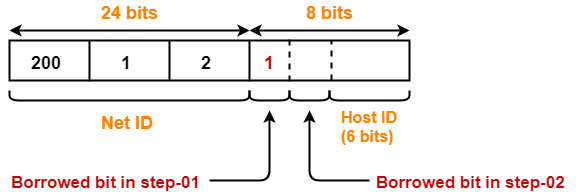• If 2nd borrowed bit = 0, then it represents one subnet.
• If 2nd borrowed bit = 1, then it represents the other subnet.

IP Address of the two subnets are-

• 200.1.2.10000000 = 200.1.2.128
• 200.1.2.11000000 = 200.1.2.192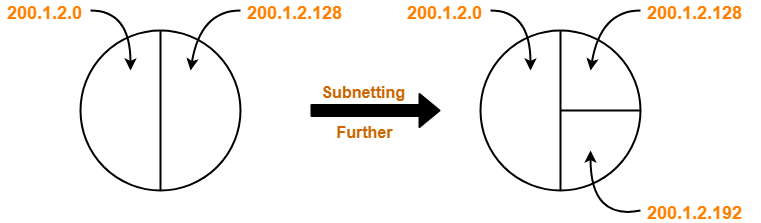Finally, the given single network is divided into 3 subnets having IP Address-

• 200.1.2.0
• 200.1.2.128
• 200.1.2.192

## For 1st Subnet-

• IP Address of the subnet = 200.1.2.0
• Total number of IP Addresses = 27 = 128
• Total number of hosts that can be configured = 128 – 2 = 126
• Range of IP Addresses = [200.1.2.00000000, 200.1.2.01111111] = [200.1.2.0, 200.1.2.127]
• Direct Broadcast Address = 200.1.2.01111111 = 200.1.2.127

## For 2nd Subnet-

• IP Address of the subnet = 200.1.2.128
• Total number of IP Addresses = 26 = 64
• Total number of hosts that can be configured = 64 – 2 = 62
• Range of IP Addresses = [200.1.2.10000000, 200.1.2.10111111] = [200.1.2.128, 200.1.2.191]
• Direct Broadcast Address = 200.1.2.10111111 = 200.1.2.191

## For 3rd Subnet-

• IP Address of the subnet = 200.1.2.192
• Total number of IP Addresses = 26 = 64
• Total number of hosts that can be configured = 64 – 2 = 62
• Range of IP Addresses = [200.1.2.11000000, 200.1.2.11111111] = [200.1.2.192, 200.1.2.255]
• Direct Broadcast Address = 200.1.2.11111111 = 200.1.2.255

## Point-01:

 Subnetting leads to loss of IP Addresses.

During subnetting,

• We have to face a loss of IP Addresses.
• This is because two IP Addresses are wasted for each subnet.
• One IP address is wasted for its network address.
• Other IP Address is wasted for its direct broadcasting address.

## Point-02:

 Subnetting leads to complicated communication process.

After subnetting, the communication process becomes complex involving the following 4 steps-

1. Identifying the network
2. Identifying the sub network
3. Identifying the host
4. Identifying the process

## Problem-01:

Suppose a network with IP Address 192.16.0.0. is divided into 2 subnets, find number of hosts per subnet.

Also for the first subnet, find-

2. First Host ID
3. Last Host ID

## Solution-

• Given IP Address belongs to class C.
• So, 24 bits are reserved for the Net ID.
• The given network is divided into 2 subnets.
• So, 1 bit is borrowed from the host ID part for the subnet IDs.
• Then, Number of bits remaining for the Host ID = 7.
• Thus, Number of hosts per subnet = 27 = 128.

### For 1st Subnet-

• Subnet Address = First IP Address = 192.16.0.00000000 = 172.16.0.0
• First Host ID = 192.16.0.00000001 = 192.16.0.1
• Last Host ID = 192.16.0.01111110 = 192.16.0.126
• Broadcast Address = Last IP Address = 192.16.0.01111111 = 172.16.0.127

## Problem-02:

What is not true about subnetting?

1. It is applied for a single network
2. It is used to improve security
3. Bits are borrowed from network portion
4. Bits are borrowed from Host portion

## Solution-

Clearly, Option (C) is correct.

## Problem-03:

In a class B, network on the internet has a subnet mask of 255.255.240.0. What is the maximum number of hosts per subnet?

1. 4096
2. 4094
3. 4092
4. 4090

## Solution-

• Number of bits reserved for network ID in the given subnet mask = 20.
• So, Number of bits reserved for Host ID = 32 – 20 = 12 bits.
• Thus, Number of hosts per subnet = 212 – 2 = 4094.
• In class B, 16 bits are reserved for the network.
• So, Number of bits reserved for subnet ID = 20 – 16 = 4 bits.
• Number of subnets possible = 24 = 16.
• Thus, Option (B) is correct.

To gain better understanding about IPv4 Subnetting,

Watch this Video Lecture

Next Article- Subnet Mask | Calculating Subnet Mask

Get more notes and other study material of Computer Networks.

Watch video lectures by visiting our YouTube channel LearnVidFun.

SummaryArticle Name
Subnetting in Networking | Subnetting Examples
Description
Subnetting in Networking is a process of dividing a single network into sub networks called as subnets. Subnetting Examples. IPv4 Subnetting Types- Fixed Length Subnetting and Variable Length Subnetting.
Author
Publisher Name
Gate Vidyalay
Publisher Logo